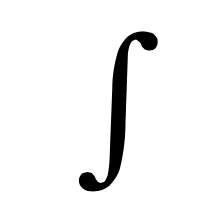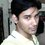# Help me to understand this integration.Consider this integral $$\displaystyle I=\int { \tan { x } \sec ^{ 2 }{ x } } dx$$.

I solved it in this way $let,\quad \sec { x } =s\\ then,\quad \tan { x } \sec { x } dx=ds\\ \therefore I=\int { s } ds=\cfrac { { s }^{ 2 } }{ 2 } +c=\cfrac { \sec ^{ 2 }{ x } }{ 2 } +c$

is it wrong?

Because my book had this solution: $I=\int { \tan { x } \sec ^{ 2 }{ x } } dx\\ let,\quad \tan { x } =t\\ then,\quad \sec ^{ 2 }{ x } dx=dt\\ I=\int { t } dt=\cfrac { { t }^{ 2 } }{ 2 } +c=\cfrac { \tan ^{ 2 }{ x } }{ 2 } +c$

How come?

Update!

Ok, I got it thanx Brilliant community.Note by Soumo Mukherjee
6 years, 7 months ago

This discussion board is a place to discuss our Daily Challenges and the math and science related to those challenges. Explanations are more than just a solution — they should explain the steps and thinking strategies that you used to obtain the solution. Comments should further the discussion of math and science.

When posting on Brilliant:

• Use the emojis to react to an explanation, whether you're congratulating a job well done , or just really confused .
• Ask specific questions about the challenge or the steps in somebody's explanation. Well-posed questions can add a lot to the discussion, but posting "I don't understand!" doesn't help anyone.
• Try to contribute something new to the discussion, whether it is an extension, generalization or other idea related to the challenge.

MarkdownAppears as
*italics* or _italics_ italics
**bold** or __bold__ bold
- bulleted- list
• bulleted
• list
1. numbered2. list
1. numbered
2. list
Note: you must add a full line of space before and after lists for them to show up correctly
paragraph 1paragraph 2

paragraph 1

paragraph 2

[example link](https://brilliant.org)example link
> This is a quote
This is a quote
    # I indented these lines
# 4 spaces, and now they show
# up as a code block.

print "hello world"
# I indented these lines
# 4 spaces, and now they show
# up as a code block.

print "hello world"
MathAppears as
Remember to wrap math in $$ ... $$ or $ ... $ to ensure proper formatting.
2 \times 3 $2 \times 3$
2^{34} $2^{34}$
a_{i-1} $a_{i-1}$
\frac{2}{3} $\frac{2}{3}$
\sqrt{2} $\sqrt{2}$
\sum_{i=1}^3 $\sum_{i=1}^3$
\sin \theta $\sin \theta$
\boxed{123} $\boxed{123}$

Sort by:

Both of the approaches here are absolutely correct. It's just that the $c$'s are different.

If the constants of integration in the first approach and the second approach are $c_1$ and $c_2$ respectively, we have $c_1+\frac{1}{2}=c_2$.

Does this help?

- 6 years, 7 months ago

So, beacuse of the constant will the definite integral be different?

- 6 years, 7 months ago

Uh no. Because the difference in the constants get cancelled out on a definite integral. :-0 o_O

So if it was something like $\int _{ a }^{ b }{ tanx{ sec }^{ 2 }x\quad dx } = \frac{ {sec}^{2}b}{2 } + { c }_{ 1 } - \frac{ {sec}^{2}a}{2 } - { c }_{ 1 }$ and similar thing will happen with $\frac{{tan}^2{x}}{2} + {c}_{2}$.

And because the difference between $\frac{{tan}^{2}x}{2}$ and $\frac{{sec}^{2}x}{2}$ is a constant. The answer will be the same.

- 6 years, 7 months ago

Yo! :P If you're curious, feel free to check out Trevor's note on this topic! :D

- 6 years, 7 months ago

i will look into the note.

- 6 years, 7 months ago

Both answers absolutely correct since, adding 1/2 to second answer (TANx^2) and subtracting 1/2 from c won't matter...

- 6 years, 7 months ago

both answers are ultimately the same. from the infinite set of c if you take out 0.5 and add it to the answer given in the book, it leads to the answer you found out! As simple as that!

- 6 years, 7 months ago

What is equal to i^2n+2

- 6 years, 7 months ago

If 'i' here is considered as the imaginary number then the value depends strictly on n. For n $in$ Z (the set of integers), the value will alternate between -1 and 1.

- 6 years, 6 months ago

The solution given in the book is right

- 6 years, 7 months ago

the c in the book method will be 1/2 less than the c in your method

- 6 years, 6 months ago

i dont understand the formula

- 6 years, 6 months ago